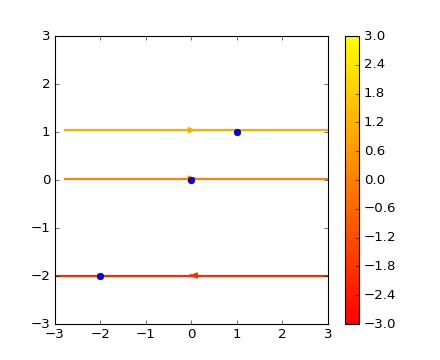### We're updating the default styles for Matplotlib 2.0

Learn what to expect in the new updatesTravis-CI:# images_contours_and_fields example code: streamplot_demo_start_points.py¶```"""
Demo of the `streamplot` function.

A streamplot, or streamline plot, is used to display 2D vector fields. This
example shows a few features of the stream plot function:

* Varying the color along a streamline.
* Varying the density of streamlines.
* Varying the line width along a stream line.
"""
import numpy as np
import matplotlib.pyplot as plt
plt.ion()

X, Y = (np.linspace(-3, 3, 100),
np.linspace(-3, 3, 100))

U, V = np.mgrid[-3:3:100j, 0:0:100j]

seed_points = np.array([[-2, 0, 1], [-2, 0, 1]])

fig0, ax0 = plt.subplots()
strm = ax0.streamplot(X, Y, U, V, color=U, linewidth=2,
cmap=plt.cm.autumn, start_points=seed_points.T)
fig0.colorbar(strm.lines)

ax0.plot(seed_points, seed_points, 'bo')

ax0.axis((-3, 3, -3, 3))
```

Keywords: python, matplotlib, pylab, example, codex (see Search examples)